# How do you generate text using a hMM model given below:One possible interpretation of the latent variables in the HMM model is that they are POS tags. We will go with this interpretation for simplicity, though the latent states could mean other things as well.

To generate text from a HMM, we need to know the transition matrix (the probability of going from one tag to another) and the emission/output matrix (the probability of generating a token given the tag.) Given this :

• First generate the state (tag)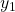.
• We then generate all the other tags using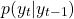.

• Then from each tag, generate a word(at each position) using the distribution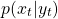. Note that this is possible because given the current tag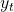, observed variable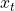doesn’t depend on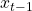and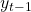.